## 结构冲击畸变问题的直接相似方法研究 1)

*西北工业大学航空学院计算力学与工程应用研究所, 西安 710072

## A DIRECT SCALING METHOD FOR THE DISTORTION PROBLEMS OF STRUCTURAL IMPACT 1)

Wang Shuai*, Xu Fei,*,2), Dai Zhen*, Liu Xiaochuan, Li Xiaocheng*, Yang leifeng*, Xi Xulong

*Institute for Computational Mechanics and Its Applications, School of Aeronautics, Northwestern Polytechnical University, Xi'an 710072, China

Aviation Key Laboratory of Science and Technology on Structures Impact Dynamics, Aircraft Strength Research Institute of China, Xi'an 710065, China

 基金资助: 1)国家自然科学基金项目.  11972309高等学校学科创新引智计划(111计划).  BP0719007

Received: 2019-11-27   Accepted: 2020-02-28   Online: 2020-05-18Abstract

The distortion of structural impact arising from the use of different materials between the full-size protype and the small-size scaled model is usually compensated by the correction for the velocity or density of the scaled model. However, the traditional correction methods are limited for the application of similarity laws because of some inherent defects such as the needs to test structural response in advance, the dependence for the special constitutive equation and the inability to reflect dynamic process of impact. In the present paper, a direct scaling method for distortion problems is proposed. Based on the best approximation relationships between the predicted flow yield stress of the scaled model and the flow yield stress of the prototype on strain rate interval, the scaling factor for the corrected velocity or the corrected density are obtained directly, and the dynamic similarity relations between the scaled model and the prototype are established. Based on the Norton-Hoff, Cowper-Symonds and Johnson-Cook constitutive models, the influences of material parameters of the strain-rate-sensitive, the reference strain-rate, the yield stress and the density for the dynamic similarity relations are studied. And a crooked plate under impact mass is used to verify the effectiveness of the proposed directly scaling method. The analysis shows that the proposed directly scaling method does not need to test the structural response information in advance, does not depend on the specific form of constitutive equation and emphasis the dynamic similarity characteristics, so that it has the direct, efficient and universal characteristics. The optimal approximation effects of the dynamic similarity relations are mainly controlled by the material strain-rate-sensitive characteristic parameters, while the reference strain-rate, the yield stress and the density have little influence. When the material strain-rate-sensitive characteristic parameters of the scale model are similar to those of the prototype, the best dynamic approximation effects can be obtained.

Keywords： similarity ; scaling ; strain-rate-sensitivity ; different materials ; impact

Wang Shuai, Xu Fei, Dai Zhen, Liu Xiaochuan, Li Xiaocheng, Yang leifeng, Xi Xulong. A DIRECT SCALING METHOD FOR THE DISTORTION PROBLEMS OF STRUCTURAL IMPACT 1). Chinese Journal of Theoretical and Applied Mechanics[J], 2020, 52(3): 774-786 DOI:10.6052/0459-1879-19-327

## 1 直接相似方法

### 1.1 传统的相似方法

$\beta_V =\sqrt {\beta_{\sigma_{d} } } =\sqrt {\frac{\left( {\sigma_{d} } \right)_{m} }{\left( {\sigma_{d} } \right)_{p} }} =\sqrt {\frac{f\left( {\dot{{\varepsilon }}_{m}^{c} } \right)}{f\left( {\dot{{\varepsilon }}_{p} } \right)}} =\sqrt{\frac{f\left( {\beta_V \dot{{\varepsilon }}_{m}^{nc} } \right)}{f\left( {\beta \dot{{\varepsilon }}_{m}^{nc} } \right)}}$

$\beta_{\sigma_{d} } =\frac{\left( {\sigma_{d} } \right)_{m} }{\left( {\sigma _{d} } \right)_{p} }=\frac{\sigma_0 \left( {\dot{{\varepsilon }}_{m} /\dot{{\varepsilon }}_0 } \right)^q}{\sigma_0 \left( {\dot{{\varepsilon }}_{p} /\dot{{\varepsilon }}_0 } \right)^q}=\left( {\beta_{\dot{{\varepsilon }}} } \right)^q =\\ \qquad\left( {\frac{\beta_V }{\beta }} \right)^q=\beta_V^2 \to \beta_V =\beta ^{q/\left( {q-2} \right)}$

Mazzariol等考虑材料应变率敏感性效应、屈服应力、密度不同引起的不同材料的畸变, 定义速度因子为

$\beta_V =\sqrt {\frac{\beta_{\sigma_0 } \beta_{visco}}{\beta_\rho }}$

### 1.2 直接相似方法的推导

$\beta_{\sigma_{d} } =\frac{\left( {\sigma_{d} } \right)_{m} }{\left( {\sigma_{d} } \right)_{p} }=\beta_\rho \beta_V^2 \to \left( {\sigma_{d} } \right)_{p} =\left( {\sigma_{d} } \right)_{m} \frac{1}{\beta_\rho \beta_V^2 }$

$\beta_{\dot{{\varepsilon }}} =\frac{\dot{{\varepsilon }}_{m} }{\dot{{\varepsilon }}_{p} }=\frac{\beta_V }{\beta }\to \dot{{\varepsilon }}_{m} =\dot{{\varepsilon }}_{p} \frac{\beta_V }{\beta }$

$\left( {\sigma_{d}' } \right)_{p} =\left( {\sigma_{d} } \right)_{m} \frac{1}{\beta_\rho \beta_V^2 }=f_{m} \left( {\dot{{\varepsilon }}_{m} } \right)\frac{1}{\beta_\rho \beta_V^2 } =\\ \qquad f_{m} \left( {\dot{{\varepsilon }}_{p} \frac{\beta_V }{\beta }} \right)\frac{1}{\beta_\rho \beta_V^2 }$

$\left\| {f_{m} \left( {\dot{{\varepsilon }}_{p} \frac{\beta_V^* }{\beta }} \right)\frac{1}{\beta_\rho \beta_V^{*2}}-f_{p} \left( {\dot{{\varepsilon }}_{p} } \right)} \right\|_2 = \\ \qquad\mathop {\min }\limits_{\beta_V \in {\mathbb{R}}} \left\| {f_{m} \left( {\dot{{\varepsilon }}_{p} \frac{\beta_V }{\beta }} \right)\frac{1}{\beta _\rho \beta_V^2 }-f_{p} \left( {\dot{{\varepsilon }}_{p} } \right)} \right\|_2$

### 图1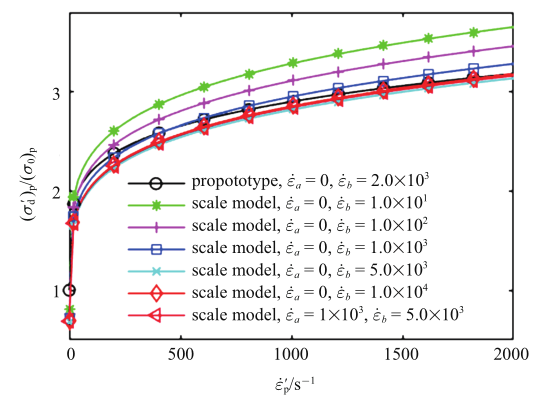Fig.1   Comparison of the prediction results for the function curves between the flow yield stress and the strain rate curves by the different strain rate intervals

### 1.3 考虑材料参数后的直接相似方法

(1)当使用Norton-Hoff本构, 根据式(7)得到

$\left\| {\left( {\sigma_0 } \right)_{m} \left( {\frac{\dot{{\varepsilon }}_{p} }{\left( {\dot{{\varepsilon }}_0 } \right)_{m} }\frac{\beta _V^* }{\beta }} \right)^{q_{m} }\frac{1}{\beta_\rho \beta_V^{*2}}-\left( {\sigma_0 } \right)_{p} \left( {\frac{\dot{{\varepsilon }}_{p} }{\left( {\dot{{\varepsilon }}_0 } \right)_{p} }} \right)^{q_{p} }} \right\|_2 =\\ \qquad\mathop {\min }\limits_{\beta_V \in {\mathbb{R}}} \left\| {\left( {\sigma_0 } \right)_{m} \left( {\frac{\dot{{\varepsilon }}_{p} }{\left( {\dot{{\varepsilon }}_0 } \right)_{m} }\frac{\beta_V }{\beta }} \right)^{q_{m} }\frac{1}{\beta_\rho \beta_V^2 }-\left( {\sigma_0 } \right)_{p} \left( {\frac{\dot{{\varepsilon }}_{p} }{\left( {\dot{{\varepsilon }}_0 } \right)_{p} }} \right)^{q_{p} }} \right\|_2$

$\left\| {\left( {\sigma_0 } \right)_{m} \left( {\frac{\dot{{\varepsilon }}_{p} }{\left( {\dot{{\varepsilon }}_0 } \right)_{m} }\frac{\beta _V^* }{\beta }} \right)^{q_{m} }\frac{1}{\beta_\rho \beta_V^{*2}}-\left( {\sigma_0 } \right)_{p} \left( {\frac{\dot{{\varepsilon }}_{p} }{\left( {\dot{{\varepsilon }}_0 } \right)_{p} }} \right)^{q_{p} }} \right\|_2 =\\ \quad\mathop {\min }\limits_{\beta_V \in {\mathbb{R}}} \left\| {\left( {\sigma_0 } \right)_{p} \left( {\frac{\dot{{\varepsilon }}_{p} }{\left( {\dot{{\varepsilon }}_0 } \right)_{p} }\frac{\beta_V }{\beta \beta _{\dot{{\varepsilon }}_0 } }} \right)^{q_{p} \beta_q }\frac{\beta_{\sigma_0 } }{\beta_\rho \beta_V^2 }-\left( {\sigma_0 } \right)_{p} \left( {\frac{\dot{{\varepsilon }}_{p} }{\left( {\dot{{\varepsilon }}_0 } \right)_{p} }} \right)^{q_{p} }} \right\|_2$

$\left\| {\left( {\sigma_0 } \right)_{m} \left( {\frac{\dot{{\varepsilon }}_{p} }{\left( {\dot{{\varepsilon }}_0 } \right)_{m} }\frac{\beta _V^* }{\beta }} \right)^{q_{m} }\frac{1}{\beta_\rho \beta_V^{*2}}-\left( {\sigma_0 } \right)_{p} \left( {\frac{\dot{{\varepsilon }}_{p} }{\left( {\dot{{\varepsilon }}_0 } \right)_{p} }} \right)^{q_{p} }} \right\|_2 =\\ \qquad \left\| {\left( {\sigma_0 } \right)_{p} \left( {\frac{\dot{{\varepsilon }}_{p} }{\left( {\dot{{\varepsilon }}_0 } \right)_{p} }} \right)^{^{q_{p} }}} \right\|_2 \times \mathop {\min }\limits_{\beta_V \in {\mathbb{R}}} \left| {\left( {\frac{\beta_V }{\beta \beta _{\dot{{\varepsilon }}_0 } }} \right)^{^{q_{p} }}\frac{\beta_{\sigma_0 } }{\beta_\rho \beta_V^2 }-1} \right|$

### 图2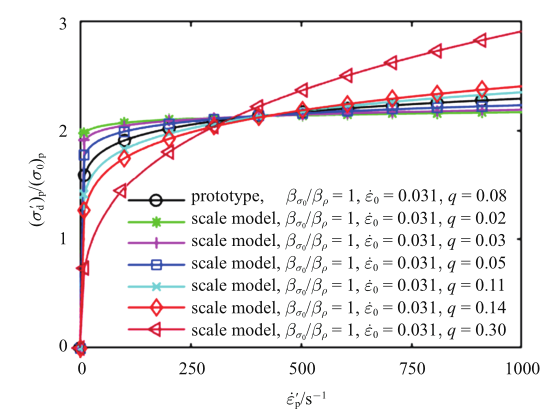Fig.2   The influence of material parameter $q$ for the function curves between the flow yield stress and the strain rate curves (Norton-Hoff equation)

(2) 当使用Cowper-Symonds本构, 根据式(7)\lb 得到

$\begin{eqnarray} \label{eq11} \left\|\left( {\sigma_0 } \right)_{m} \left[ {1+\left( {\frac{\dot{{\varepsilon }}_{p} }{D_{m} }\frac{\beta_V^* }{\beta }} \right)^{{1/P}_{m} }} \right]\frac{1}{\beta_\rho \beta_V^{*2}}-\\ \qquad\left( {\sigma_0 } \right)_{p} \left[ {1+\left( {\frac{\dot{{\varepsilon }}_{p} }{D_{p} }} \right)^{{1/P}_{p} }} \right] \right\|_2 =\\ \qquad\mathop {\min }\limits_{\beta_V \in {\mathbb{R}}} \left\|\left( {\sigma_0 } \right)_{m} \left[ {1+\left( {\frac{\dot{{\varepsilon }}_{p} }{D_{m} }\frac{\beta_V }{\beta }} \right)^{{1/P}_{m} }} \right]\frac{1}{\beta_\rho \beta_V^2 }-\\ \qquad\left( {\sigma_0 } \right)_{p} \left[ {1+\left( {\frac{\dot{{\varepsilon }}_{p} }{D_{p} }} \right)^{{1/P}_{p} }} \right] \right\|_2 \end{eqnarray}$

$\begin{eqnarray} \label{eq12} \left\| \left( {\sigma_0 } \right)_{m} \left[ {1+\left( {\frac{\dot{{\varepsilon }}_{p} }{D_{m} }\frac{\beta_V^* }{\beta }} \right)^{{1/P}_{m} }} \right]\frac{1}{\beta_\rho \beta_V^{*2}}-\\ \qquad\left( {\sigma_0 } \right)_{p} \left[ {1+\left( {\frac{\dot{{\varepsilon }}_{p} }{D_{p} }} \right)^{{1/P}_{p} }} \right] \right\|_2 =\\ \qquad \left( {\sigma_0 } \right)_{p} \times \mathop {\min }\limits_{\beta_V \in {\mathbb{R}}} \left\| \left[ {1+\left( {\frac{\dot{{\varepsilon }}_{p} }{D_{p} }\frac{\beta_V }{\beta_D \beta }} \right)^{{1/P}_{p} \beta_{p} }} \right]\frac{\beta_{\sigma_0 } }{\beta _\rho \beta_V^2 }-\\ \qquad\left[ {1+\left( {\frac{\dot{{\varepsilon }}_{p} }{D_{p} }} \right)^{{1/P}_{p} }} \right] \right\|_2 \end{eqnarray}$

### 图3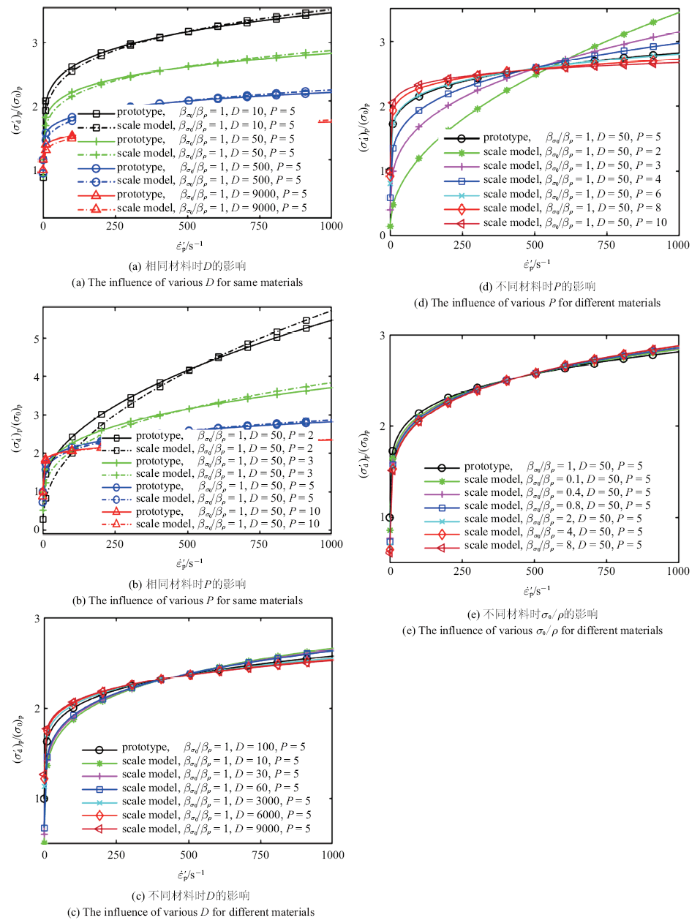Fig.3   The influence of material parameters for the function curves between the flow yield stress and the strain rate curves (Cowper-Symonds equation)

(3) 当使用简化后的Johnson-Cook本构模型(忽略应变硬化效应和温度效应), 即$\sigma _{d} =\sigma_0 [1+C\ln (\dot{{\varepsilon }}/\dot{{\varepsilon }}_0 )]$, 式中, $C$为应变率敏感性特征参数、$\dot{{\varepsilon }}_0$为参考应变率参数, 通常设定为1 s$^{-1}$. 根据式(7)得到

$\begin{eqnarray} \label{eq13} \left\| \left( {\sigma_0 } \right)_{m} \left[ {1+C_{m} \ln \left( {\frac{\dot{{\varepsilon }}_{p} }{\left( {\dot{{\varepsilon }}_0 } \right)_{m} }\frac{\beta_V^* }{\beta }} \right)} \right]\frac{1}{\beta_\rho \beta _V^{*2}}-\\ \lt.\qquad\left( {\sigma_0 } \right)_{p} \left[ {1+C_{p} \ln \left( {\frac{\dot{{\varepsilon }}_{p} }{\left( {\dot{{\varepsilon }}_0 } \right)_{p} }} \right)} \right] \right\|_2 =\\ \qquad \mathop {\min }\limits_{\beta_V \in {\mathbb{R}}} \left\| \left( {\sigma_0 } \right)_{m} \left[ {1+C_{m} \ln \left( {\frac{\dot{{\varepsilon }}_{p} }{\left( {\dot{{\varepsilon }}_0 } \right)_{m} }\frac{\beta_V }{\beta }} \right)} \right]\frac{1}{\beta_\rho \beta_V^2 }-\\ \\qquad\left( {\sigma_0 } \right)_{p} \left[ {1+C_{p} \ln \left( {\frac{\dot{{\varepsilon }}_{p} }{\left( {\dot{{\varepsilon }}_0 } \right)_{p} }} \right)} \right] \right\|_2 \end{eqnarray}$

$\begin{eqnarray} \label{eq2} \left\| \left( {\sigma_0 } \right)_{m} \left[ {1+C_{m} \ln \left( {\frac{\dot{{\varepsilon }}_{p} }{\left( {\dot{{\varepsilon }}_0 } \right)_{m} }\frac{\beta_V^* }{\beta }} \right)} \right]\frac{1}{\beta_\rho \beta _V^{*2}}-\\ \qquad\left( {\sigma_0 } \right)_{p} \left[ {1+C_{p} \ln \left( {\frac{\dot{{\varepsilon }}_{p} }{\left( {\dot{{\varepsilon }}_0 } \right)_{p} }} \right)} \right] \right\|_2 =\\ \qquad \left( {\sigma_0 } \right)_{p} \times \mathop {\min }\limits_{\beta_V \in {\mathbb{R}}} \left\| \left[ {1+\beta_C C_{p} \ln \left( {\frac{\dot{{\varepsilon }}_{p} }{\left( {\dot{{\varepsilon }}_0 } \right)_{p} }\frac{\beta_V }{\beta \beta_{\dot{{\varepsilon }}_0 } }} \right)} \right]\frac{\beta_{\sigma_0 } }{\beta_\rho \beta_V^2 }-\\ \qquad\left[ {1+C_{p} \ln \left( {\frac{\dot{{\varepsilon }}_{p} }{\left( {\dot{{\varepsilon }}_0 } \right)_{p} }} \right)} \right] \right\|_2 \end{eqnarray}$

### 图4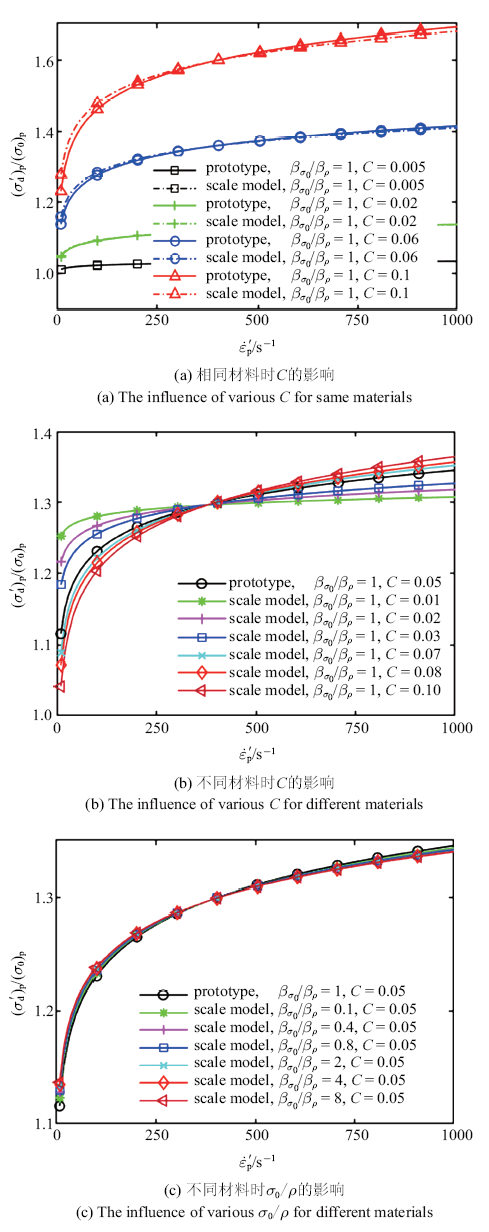Fig.4   The influence of material parameters for the function curves between the flow yield stress and the strain rate curves (Johnson-Cook equation)

$\begin{eqnarray} \label{eq15} \hspace{-4mm} \left\| {f_{m} \left( {\dot{{\varepsilon }}_{p} \frac{\beta_V^* }{\beta },X_{p} \beta_X^* } \right)\frac{1}{\beta_\rho \beta_V^{*2}}-f_{p} \left( {\dot{{\varepsilon }}_{p} ,X_{p} } \right)} \right\|_2 = \\ \hspace{-4mm}\qquad \mathop {\min }\limits_{\beta_V ,\beta_X \in {\mathbb{R}}}\left\| {f_{m} \left( {\dot{{\varepsilon }}_{p} \frac{\beta_V }{\beta },X_{p} \beta_X } \right)\frac{1}{\beta_\rho \beta_V^2 }-f_{p} \left( {\dot{{\varepsilon }}_{p} ,X_{p} } \right)} \right\|_2 \end{eqnarray}$

### 图5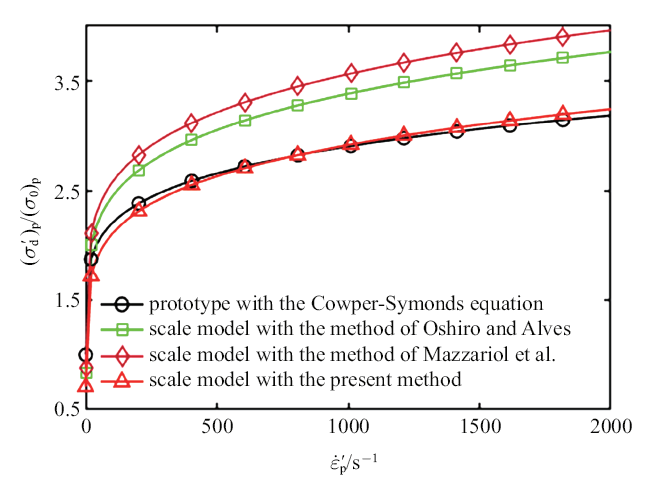Fig.5   Comparison of the prediction results for the function curves between the flow yield stress and the strain rate curves by different scaling methods

## 2 数值算例验证

### 图6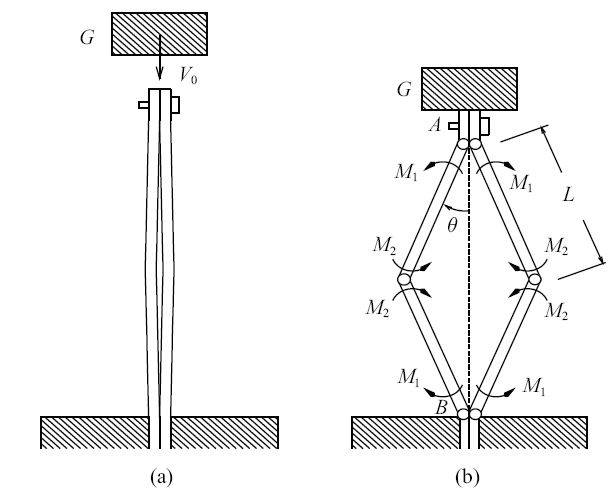Fig.6   Crooked plates under axial impact. (a) First phase of motion, (b) second phase of motion

$V_0 -\frac{2N_{p} }{G}t=\frac{\gamma w_0^2 }{L}\sinh \left({2\gamma t} \right)+\dot{c}$

$\theta_{1} =\frac{w_0 }{L}\cosh \left( {\gamma t} \right)$
$\dot{{\theta }}_{1} =\frac{w_0 }{L}\gamma \sinh \left( {\gamma t} \right)$

Zhang 和 Yu给出了第二阶段的运动方程

$\ddot{{\theta }}+\frac{L^2\left( {m+G} \right)\left( {\sin \theta } \right)\left( {\cos \theta } \right)\dot{{\theta }}^2+\left( {M_1 +M_2 } \right)}{L^2\left[ {m/3+\left( {m+G} \right)\sin ^2\theta } \right]}=0$

$M_1 =\left[ {1+\frac{2p}{2p+1}\left( {\frac{\dot{{\theta }}}{8D}} \right)^{1/p}} \right]M_0$
$M_2 =\left[ {1+\frac{2p}{2p+1}\left( {\frac{\dot{{\theta }}}{4D}} \right)^{1/p}} \right]M_0$

### 2.2 结果分析

Table 1  Material parameters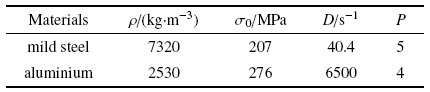Table 2  The velocity scaling factors for the various geometric crooked plates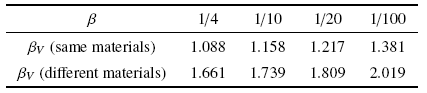### 图7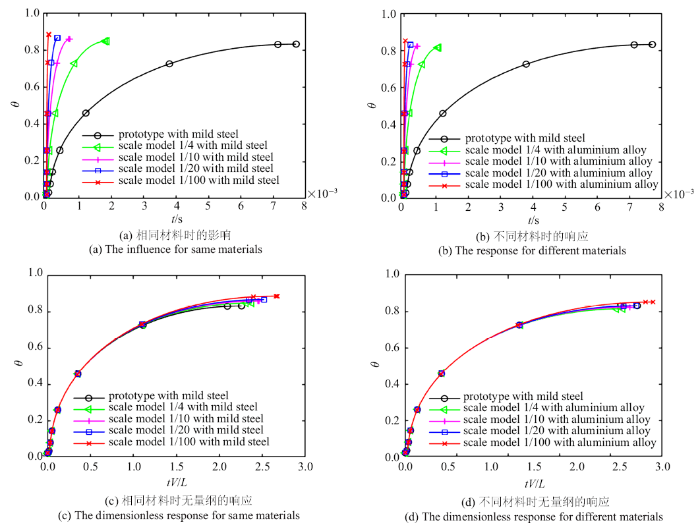Fig.7   Evolution of the rotation with movement time for the various geometric crooked plates

## 3 结论

/

 〈〉